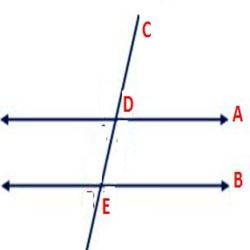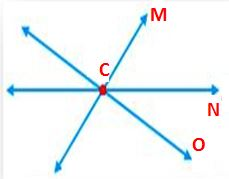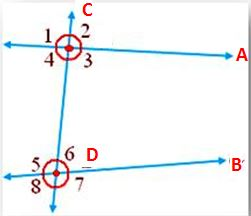# Transversal Lines – Definition, Properties, & Examples

A Transversal Line is a line that crosses at least two lines (Parallel Lines) and intersects with them. Find Problems on Transversal Lines with Solutions for a better understanding of the concept. Check concept and examples of Lines and Angles on our website for free.From the above figure, A and B are parallel lines and line C is intersecting both lines at points D and E. The line C is called as Transversal Line.From the above figure, The line M intersecting Lines N and O at a point c. But the Lines N and O are not parallel lines. Therefore, Line M is not a Transversal Line.

## Angles made by the Transversal with Two LinesFrom the above figure, Line C intersecting Line A and B (parallel lines). The angles formed around the Transversal line are called Transversal angles. From the given figure, ∠1, ∠2, ∠3, ∠4, ∠5, ∠6, ∠7, ∠8 are the angles with special names.

### Interior Angles

The angles that present in the area enclosed between two parallel lines and that are intersected by a transversal are also called interior angles. From figure, ∠3, ∠4, ∠5, ∠6 are interior angles.

### Exterior Angles

The angles that present outside two parallel lines and that are intersected by a transversal are called Exterior angles. From the figure, ∠1, ∠2, ∠7, ∠8 are Exterior angles.

### Corresponding Pair of Angles

The corresponding pair of angles present on the same side of the transversal. In the Pair of corresponding angles, if one is an interior angle, the other will be an exterior angle. Also, they do not form a linear pair. From the figure, (∠2, ∠6); (∠1, ∠5); (∠3, ∠7); (∠4, ∠8) are the Corresponding pair of angles.

### Pair of Alternate Angles

Pair of alternate angles present on the opposite sided of the transversal. Both Pair of Alternate Angles either interior angles or both are exterior angles. They will not form a linear pair. From the figure, (∠4, ∠6) and (∠3, ∠5) are interior alternate angles. Also, (∠1, ∠7) and (∠2, ∠8) are exterior alternate angles.

### Pair of Co-interior or Conjoined or Allied Angles

Pair of co-interior or Conjoined or Allied Angles are pairs of interior angles that present on the same side on the transversal. From the figure, (∠3, ∠6); (∠4, ∠5) are co-interior angles.

### Two Parallel Lines are cut by the TransversalWhen two parallel lines A and B are intersected by a Transversal line C, then

• the pairs of alternate angles are equal ∠4 = ∠6, ∠ 1 = ∠7, ∠3 =∠5, ∠2 = ∠8.
• the pairs of corresponding angles are equal ∠2 = ∠6, ∠1 = ∠5, ∠3 = ∠7, ∠4 = ∠8.
• Interior angles on the same side of transversal are supplementary ∠4 + ∠ 5 = 180°, ∠6 = 180°.

### Converse

When two parallel lines are intersected by a Transversal line

• pairs of alternate angles are equal
• pairs of corresponding angles are equal
• interior angles on the same side of the transversal are supplementary.
Scroll to Top